Examples

Chapter 3 Class 12 Matrices
Serial order wise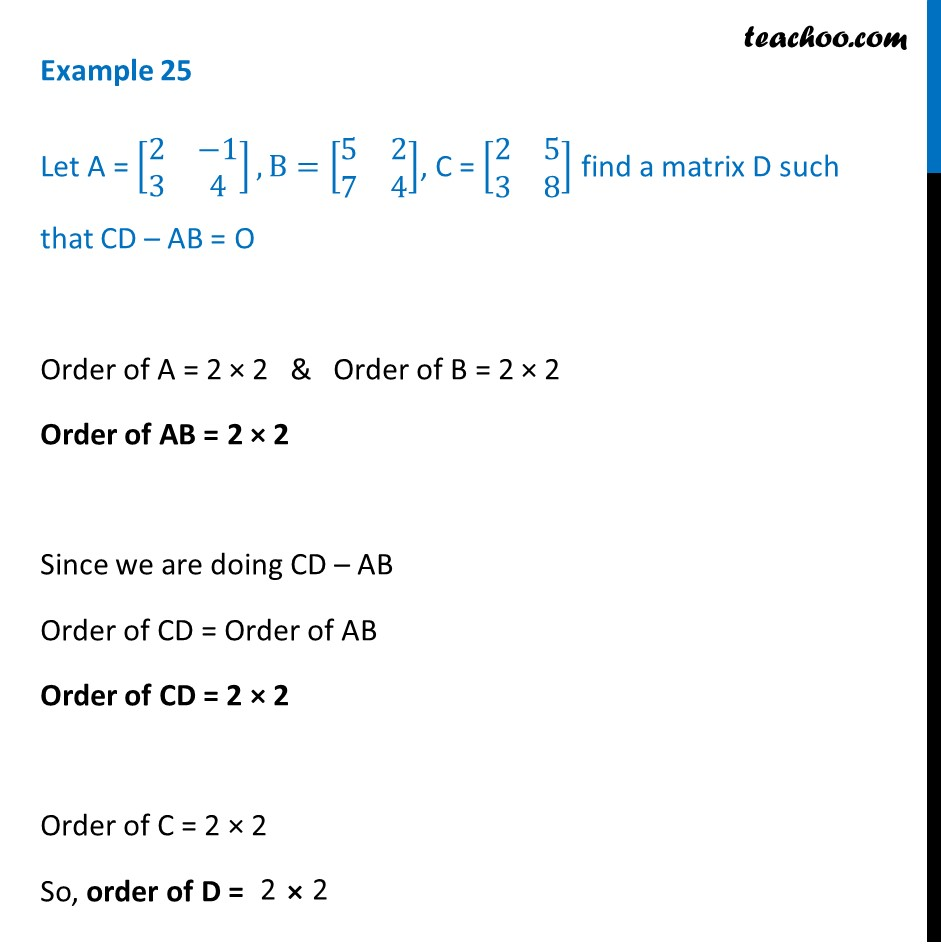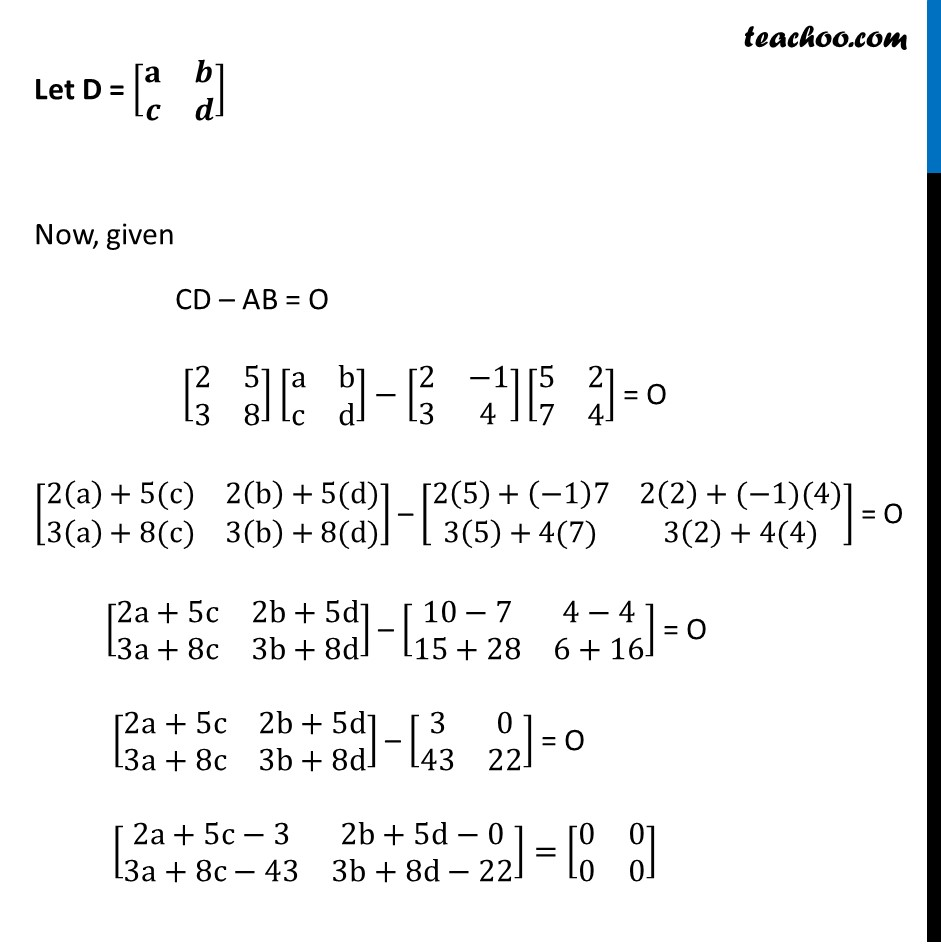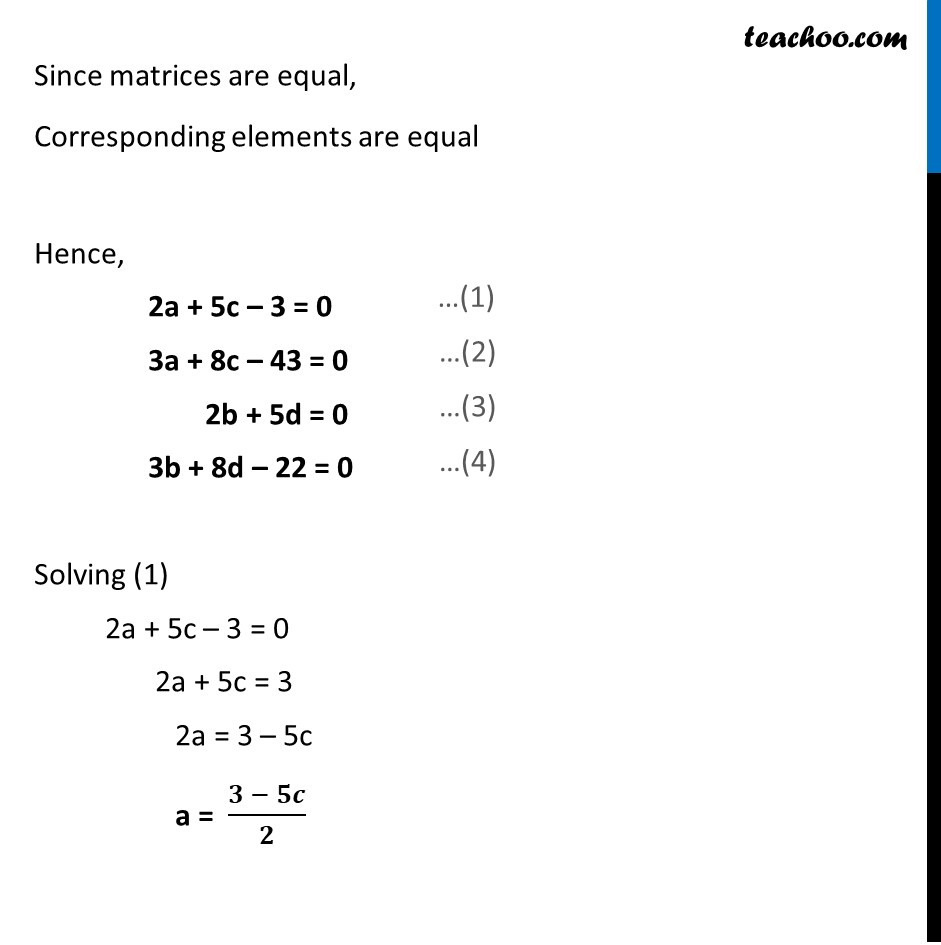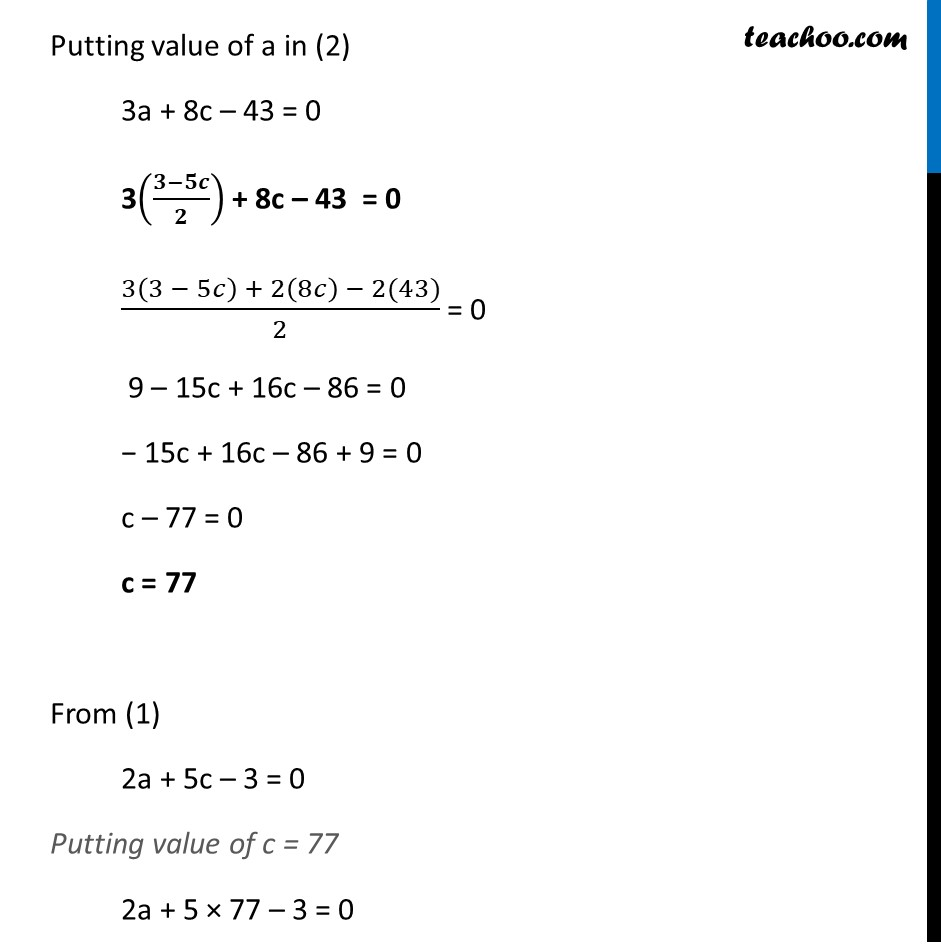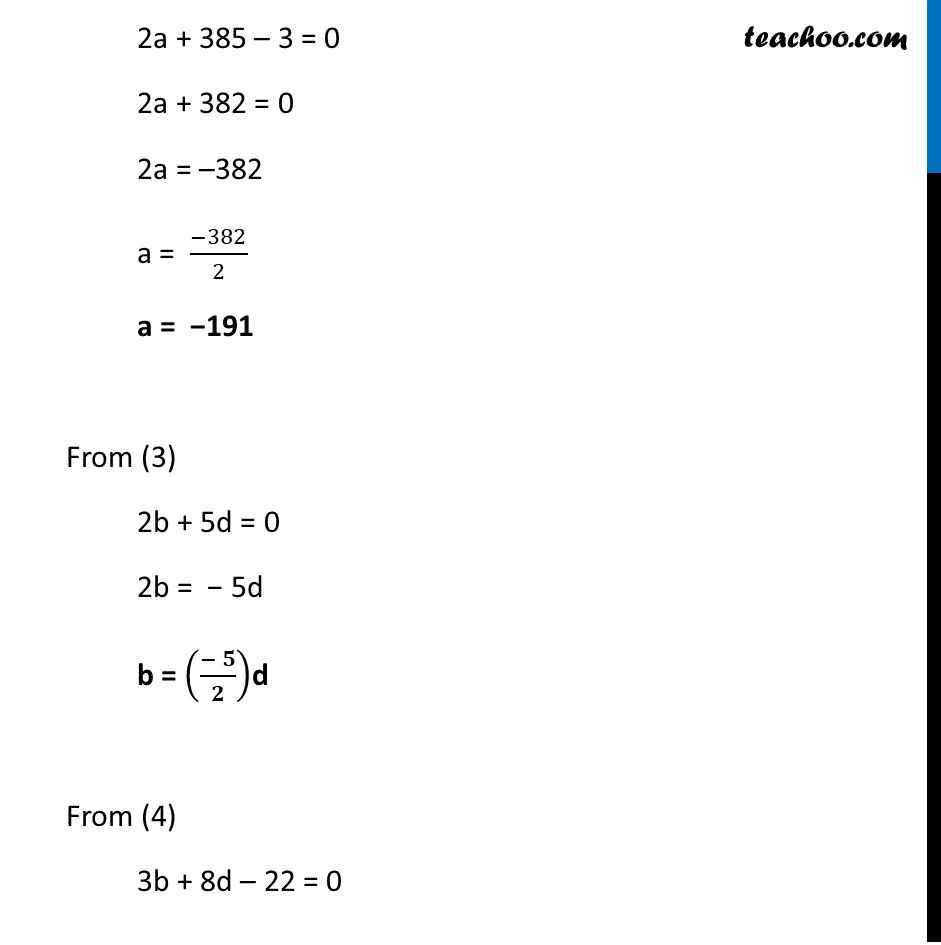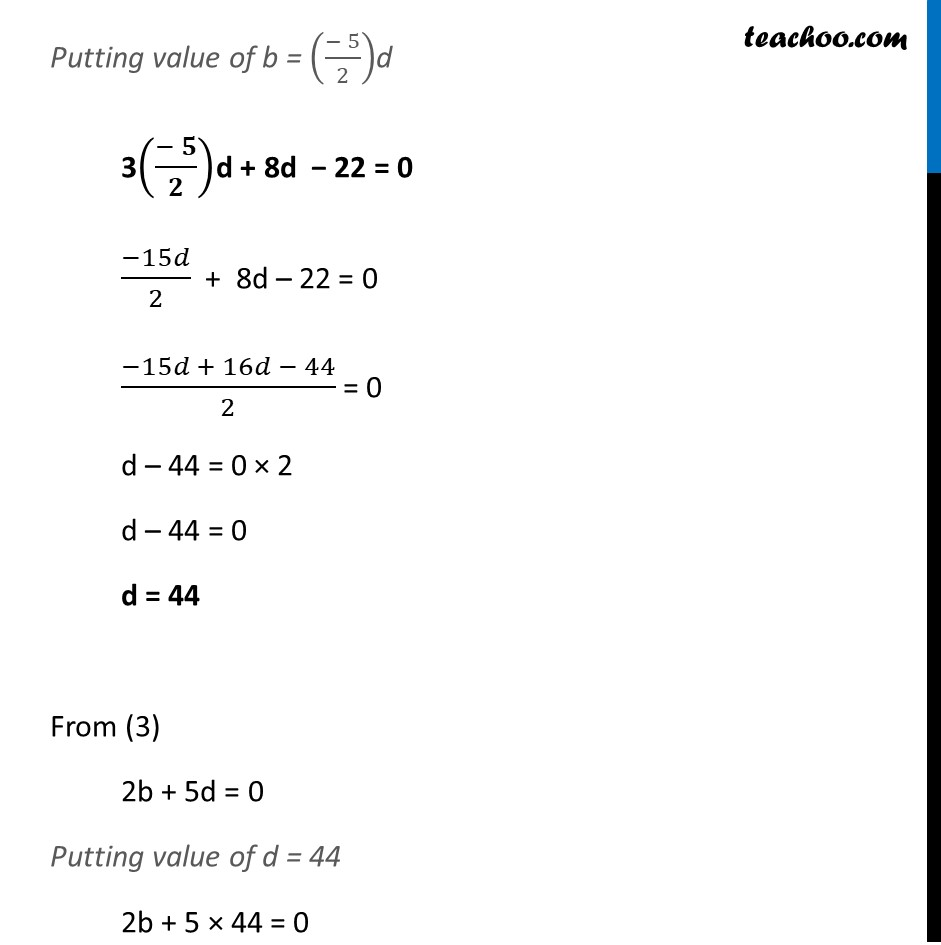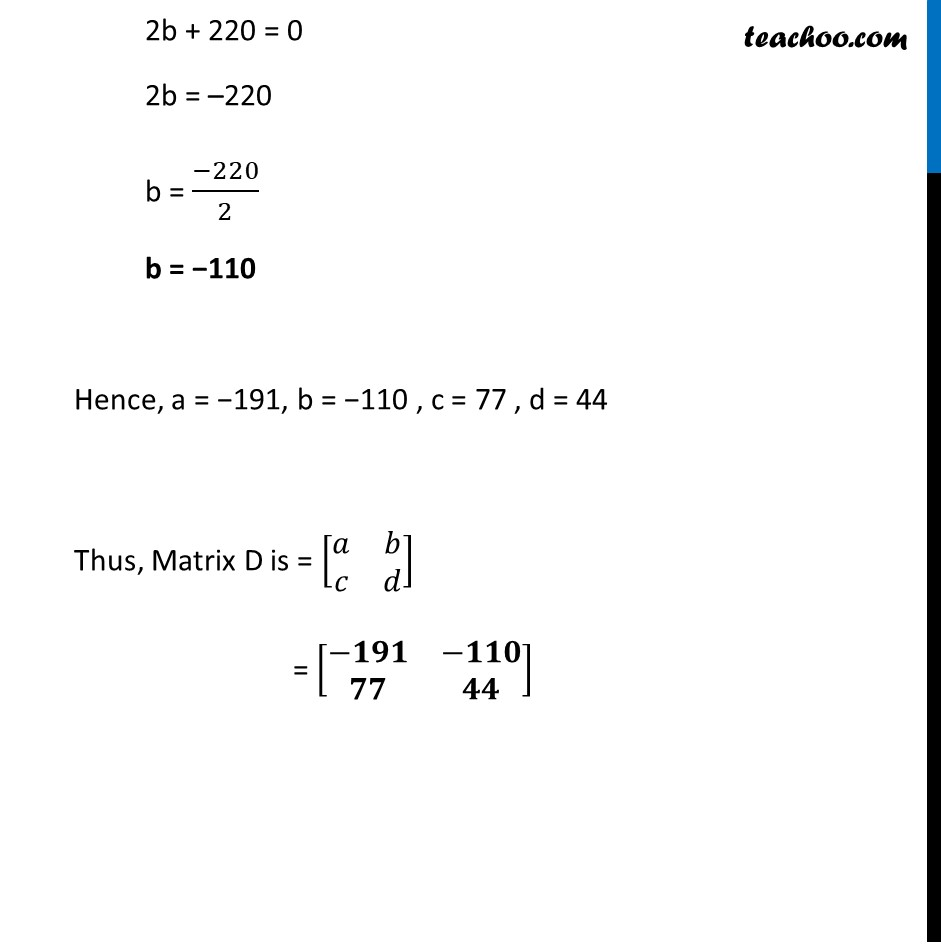Learn in your speed, with individual attention - Teachoo Maths 1-on-1 Class

### Transcript

Example 25 Let A = [■8(2&−1@3&4)], B=[■8(5&2@7&4)], C = [■8(2&5@3&8)] find a matrix D such that CD – AB = O Order of A = 2 × 2 & Order of B = 2 × 2 Order of AB = 2 × 2 Since we are doing CD – AB Order of CD = Order of AB Order of CD = 2 × 2 Order of C = 2 × 2 So, order of D = × Let D = [■8(𝐚&𝒃@𝒄&𝒅)] Now, given CD – AB = O [■8(2&5@3&8)] [■8(a&b@c&d)] − [■8(2&−1@3&4)][■8(5&2@7&4)] = O [■8(2(a)+5(c)&2(b)+5(d)@3(a)+8(c)&3(b)+8(d))] – [■8(2(5)+(−1)7&2(2)+(−1)(4)@3(5)+4(7)&3(2)+4(4))] = O [■8(2a+5c&2b+5d@3a+8c&3b+8d)] – [■8(10−7&4−4@15+28&6+16)] = O [■8(2a+5c&2b+5d@3a+8c&3b+8d)] – [■8(3&0@43&22)] = O [■8(2a+5c−3&2b+5d−0@3a+8c−43&3b+8d−22)]=[■8(0&0@0&0)] Since matrices are equal, Corresponding elements are equal Hence, 2a + 5c – 3 = 0 3a + 8c – 43 = 0 2b + 5d = 0 3b + 8d – 22 = 0 Solving (1) 2a + 5c – 3 = 0 2a + 5c = 3 2a = 3 – 5c a = (𝟑 − 𝟓𝒄)/𝟐 Putting value of a in (2) 3a + 8c – 43 = 0 3((𝟑−𝟓𝒄)/𝟐) + 8c – 43 = 0 (3(3 − 5𝑐) + 2(8𝑐) − 2(43))/2 = 0 9 – 15c + 16c – 86 = 0 − 15c + 16c – 86 + 9 = 0 c – 77 = 0 c = 77 From (1) 2a + 5c – 3 = 0 Putting value of c = 77 2a + 5 × 77 – 3 = 0 2a + 385 – 3 = 0 2a + 382 = 0 2a = –382 a = (−382)/2 a = −191 From (3) 2b + 5d = 0 2b = − 5d b = ((− 𝟓)/𝟐)d From (4) 3b + 8d – 22 = 0 Putting value of b = ((− 5)/2)d 3((− 𝟓)/𝟐)d + 8d − 22 = 0 (−15𝑑)/2 + 8d – 22 = 0 (−15𝑑 + 16𝑑 − 44)/2 = 0 d – 44 = 0 × 2 d – 44 = 0 d = 44 From (3) 2b + 5d = 0 Putting value of d = 44 2b + 5 × 44 = 0 2b + 220 = 0 2b = –220 b = (−220)/2 b = −110 Hence, a = −191, b = −110 , c = 77 , d = 44 Thus, Matrix D is = [■8(𝑎&𝑏@𝑐&𝑑)] = [■8(−𝟏𝟗𝟏&−𝟏𝟏𝟎@𝟕𝟕&𝟒𝟒)]